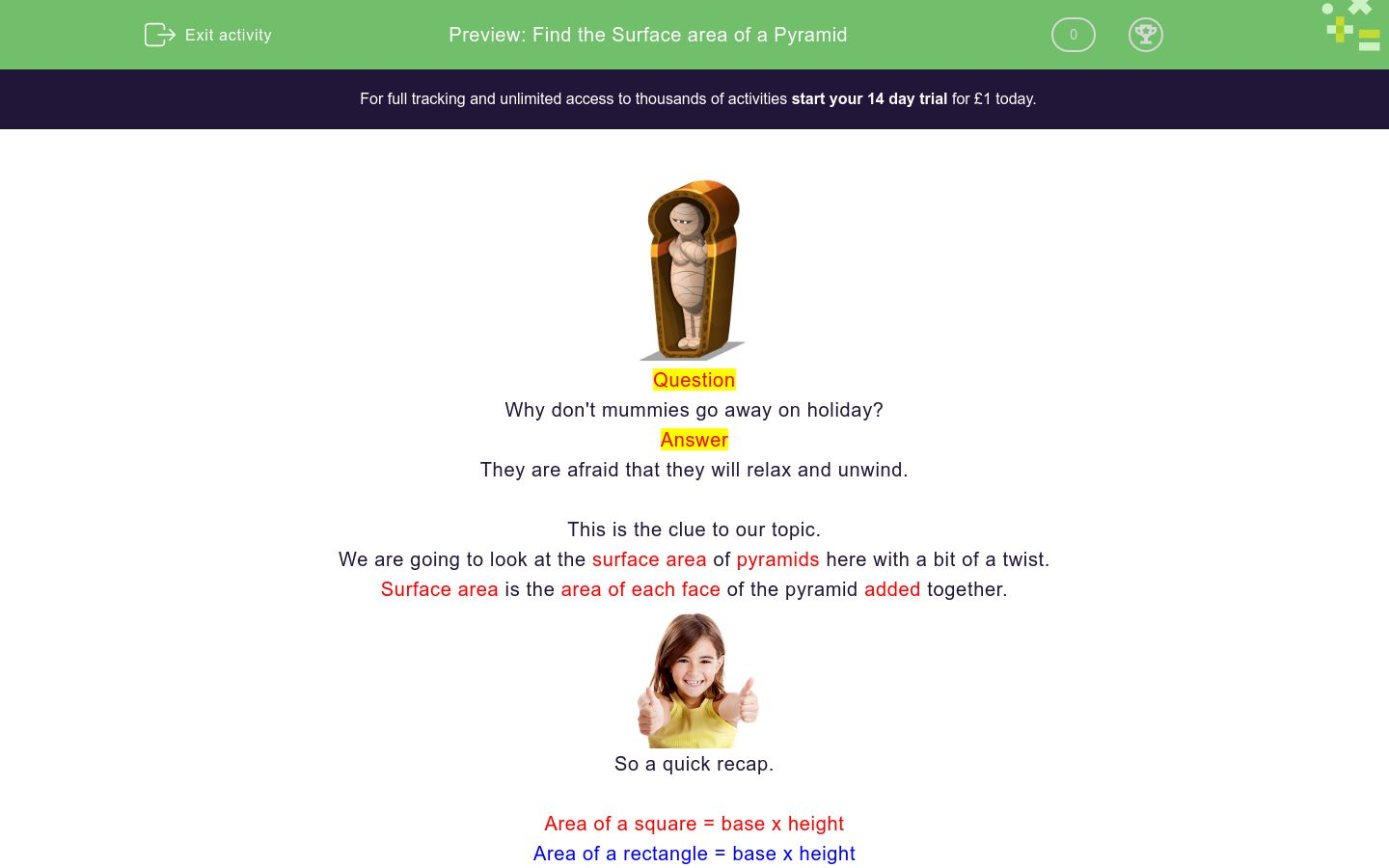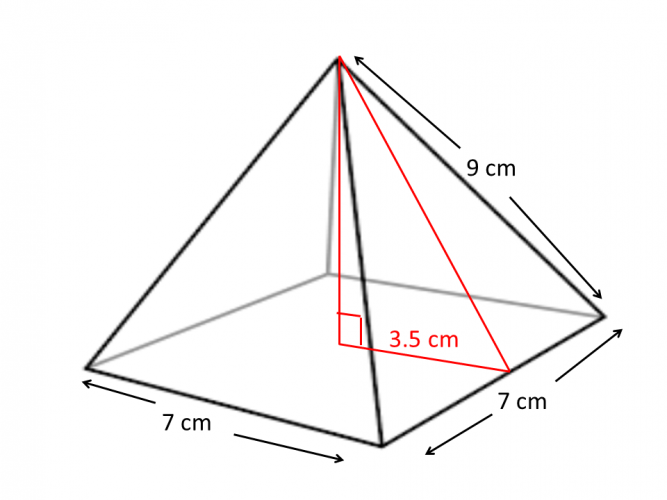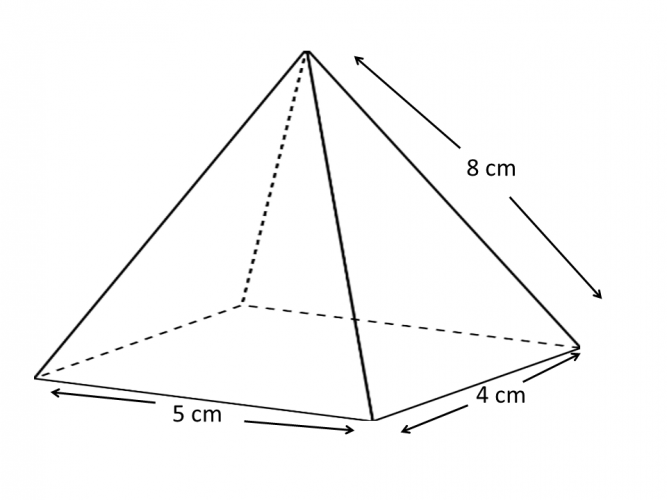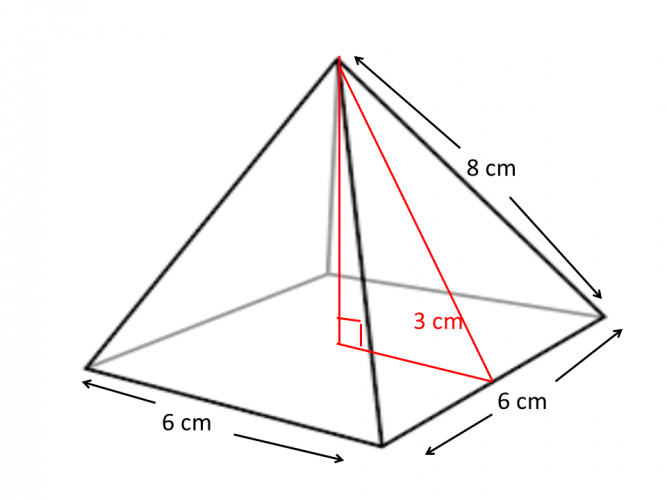# Find the Surface area of a Pyramid

In this worksheet, students will learn the formula for finding the surface area of a pyramid involving 3D trigonometry.Key stage:  KS 4

GCSE Subjects:   Maths

GCSE Boards:   AQA, Eduqas, Pearson Edexcel, OCR

Curriculum topic:   Geometry and Measures, Mensuration

Curriculum subtopic:   Mensuration and Calculation, Volume and Surface Area Calculations

Difficulty level:### QUESTION 1 of 10Question

Why don't mummies go away on holiday?

They are afraid that they will relax and unwind.

This is the clue to our topic.

We are going to look at the surface area of pyramids here with a bit of a twist.

Surface area is the area of each face of the pyramid added together.So a quick recap.

Area of a square = base x height

Area of a rectangle = base x height

Area of a triangle = base x height ÷ 2

Example 1This pyramid has a side length of 4 cm and it's height is 5 cm.

To find the surface area of this square based pyramid we

1. Calculate the area of the base  4 x 4 = 16 cm²

2. Find the area of one of the triangular sides  4 x 5 ÷ 2 = 10 cm²

3.  There are four sides to the pyramid so 10 x 4 = 40 cm²

4. Add the total  area of the sides to the area of the base =  16 + 40 = 56 cm²

Example 2  - Here is the twist - there is no height given.

Find the surface area of this square based pyramid.Don't panic you have got this.

You will need to apply 3d trigonometry (Pythagoras' Theorem) to find the height.The apex of the pyramid is exactly over the centre of the base.

1. Draw a line in vertically to the base to form a right angled triangle.

2. Apply Pythagoras' Theorem to find the height.

9 cm² - 3.5 cm² =  √68.75 cm² = 8.3 cm (rounded to 1 decimal place)

Now you can find the surface area.

To find the area of this square based pyramid we

1. Calculate the area of the base  7 x 7 = 49 cm²

2.  We find the area of the triangle with the base of 5 cm, 7 x 8.3 ÷ 2 = 29.05 cm²

3.  There are four triangles in total  29.05 x 4 = 116.2 cm²

4.  Add the base and the four triangles together 49 + 116.2  = 165.2 cm² (correct to 1 decimal place)

Find the area of this rectangular based pyramid.Example 3

This is a little different so be carefulBecause this is rectangular we don't know whether to halve 4 cm or 5 cm. Instead we apply Pythagoras' Theorem twice to be accurate.

1. Find the length of the diagonal 5 cm² + 4 cm² = √41 cm² = 6.4 cm

2. Halve 6.4 to find the mid point 6.4 cm ÷ 2 = 3.2 cm

3. Calculate the height 8 cm² - 3.2 cm⊃2 = √53.76 cm² = 7.3 cm (correct to 1 decimal place.

Surface area

1. Base = 5 x 4 = 20 cm²

2. One triangle = 5 x 7.3 ÷ 2 = 18.25 cm² (x 2 for the opposite face) = 36.5 cm²

3. The other face triangle = 4 x 7.3 ÷ 2 = 14.6 cm&sup2; (x 2 for the opposite face) = 29.2cm&sup2;

4.  Add the faces together 20 + 18.25 + 18.25 + 14.6 + 14.6 = 85.7 cm²Where do Pharaoh's like to eat?

Pizza Tut

Hopefully this has made you smile as you start the activities.

Find the surface area of this square based pyramid.195.8 cm²

213.6 cm²

177.6 cm²

124.8 cm²What is the surface area of this pyramid?

Find the surface area of this square based pyramid.Side length 9 cm  and slant height 18 cm.

313.2 cm²

394.2 cm²

626.4 cm²

542.3 cm²Calculate the surface area of this square based pyramid.

Find the surface area of this rectangular based pyramid.What is the surface area of this pyramid?208 cm² 198 cm² 118 cm² 98 cm² Surface AreaWhat is the  surface area of this pyramid.

The surface area of this pyramid is 133.76 cm² 124.4 cm²Find the surface area of this pyramid.

 566 cm² 516 cm² 536 cm² 508.6cm² Surface area

Find the surface area of this rectangular based pyramid.The surface area of this pyramid is 148.6 cm², 163.2 cm², 173.4 cm²
• Question 1

Find the surface area of this square based pyramid.124.8 cm²
EDDIE SAYSDid you remember to apply Pythagoras' Theorem first. This is the key to these types of question. Height 8 cm² - 3 cm² =√55 cm² = 7.4 cm Base area = 6 x 6 = 36 cm² Next find the area of 1 triangle 6 x 7.4 ÷ 2 = 22.2cm² Multiply the area of the triangle by 4. 22.2 x 4 = 88.8 cm² Now add this to the area of the base 88.8 + 36 = 124.8 cm² By the way, what music do mummies listen to? Rap
• Question 2What is the surface area of this pyramid?

137.6
EDDIE SAYS
Let our friend Pythagoras' do his bit first. Height 10 cm² - 4 cm² =√84 cm² = 9.2 cm Base area = 8 x 8 = 64 cm² Next find the area of 1 triangle 8 x 9.2 ÷ 2 = 18.4 cm² 18.4 x 4 = 73.6 cm² 73.6 + 64 = 137.6 cm²
• Question 3

Find the surface area of this square based pyramid.Side length 9 cm  and slant height 18 cm.

394.2 cm²
EDDIE SAYS
If in doubt sketch it out. Always. It really does help. Pythagoras' first. He never did like to be kept waiting. Height 18 cm² - 4.5 cm² =√303.75 cm² = 17.4cm Base area = 9 x 9 = 81 cm² Next find the area of 1 triangle 9 x 17.4 ÷ 2 = 78.3 cm² 78.3 x 4 = 313.2 cm² 313.2 + 81 = 394.2 cm²
• Question 4Calculate the surface area of this square based pyramid.

68.6
EDDIE SAYS
Height 10 cm² - 1.5 cm² =√97.75 cm² = 9.9 cm Base area = 3 x 3 = 9 cm² Next find the area of 1 triangle 3 x 9.9 ÷ 2 = 14.9 cm² 14.9 x 4 = 59.6 cm² 59.6 + 9 = 68.6 cm² Brilliant stuff. This is a great way to show off more than one mathematical skill.
• Question 5

Find the surface area of this rectangular based pyramid.116.6
EDDIE SAYS
Now its time to really show off. Here you have to apply Pythagoras theorem twice because we have a rectangular base. Find length of the diagonal first. 9 cm² + 7 cm² = √130 cm² = 11.4 cm 11.4 cm ÷ 2 = 5.7 cm Now we are ready to rock and roll. Height 8 cm² - 5.7 cm² =√31.51 cm² = 5.6 cm Base area = 9 x 7 = 63 cm² Next find the area of 1 triangle 9 x 5.6 ÷ 2 = 25.2 cm² Find the area of the other triangle 7 x 5.6 ÷ 2 = 19.6 cm² 63 + 25.2 + 25.2 + 19.6 + 19.6 = 116.6 cm²
• Question 6EDDIE SAYS
Find length of the diagonal first. 6 cm² + 4 cm² = √52 cm² = 7.2 cm 7.2 cm ÷ 2 =3.6 cm And we are off Height 9 cm² - 3.6 cm² =√68.04 cm² = 8.2cm Base area = 6 x 4 = 24 cm² Next find the area of 1 triangle 6 x 8.2 ÷ 2 = 24.6 cm² Find the area of the other triangle 4 x 8.2 ÷ 2 = 16.4 cm² 24 + 24.6 + 24.6 + 16.4 + 16.4 = 106 cm²
• Question 7

What is the surface area of this pyramid?208 cm² 198 cm² 118 cm² 98 cm² Surface Area
EDDIE SAYS
Find length of the diagonal first. 8 cm² + 9 cm² = √145 cm² = 12.0 cm 12.0 cm ÷ 2 = 6 cm Here we go again. Height 10 cm² - 6 cm² =√64 cm² = 8 cm Base area = 8 x 9 = 72 cm² Next find the area of 1 triangle 8 x 8 ÷ 2 = 32 cm² Find the area of the other triangle 9 x 8 ÷ 2 = 36 cm² 72 + 32 + 32+ 36 + 36 = 208 cm²
• Question 8What is the  surface area of this pyramid.

The surface area of this pyramid is 133.76 cm² 124.4 cm²
EDDIE SAYS
Find length of the diagonal first. 5 cm² + 4 cm² = √41 cm² = 6.4 cm 6.4 cm ÷ 2 = 3.2 cm Height 12 cm² - 3.2 cm² =√133.76 cm² = 11.6 cm Base area = 5 x 4 = 20 cm² Next find the area of 1 triangle 5 x 11.6 ÷ 2 = 29 cm² Find the area of the other triangle 4 x 11.6 ÷ 2 = 23.2 cm² 20+ 23.2 + 23.2 + 29 + 29 = 124.4 cm²
• Question 9Find the surface area of this pyramid.

 566 cm² 516 cm² 536 cm² 508.6cm² Surface area
EDDIE SAYS
What did the Pharaoh say when he saw the pyramid? Mummies home. Are you home and dry with these yet? Find length of the diagonal first. 14 cm² + 12 cm² = √340 cm² = 18.4 cm 18.4 cm ÷ 2 = 9.2 cm Height 16 cm² - 9.2 cm² =√171.36 cm² = 13.1 cm Base area = 14 x 12 = 168 cm² Next find the area of 1 triangle 14 x 13.1 ÷ 2 = 91.7cm² Find the area of the other triangle 12 x 13.1 ÷ 2 = 78.6 cm² 168 + 91.7 + 91.7 + 78.6 + 78.6 = 508.6 cm²
• Question 10

Find the surface area of this rectangular based pyramid.The surface area of this pyramid is 148.6 cm², 163.2 cm², 173.4 cm²
EDDIE SAYS
And the final push. 9 cm² + 5 cm² = √106 cm² = 10.3 cm 10.3 cm ÷ 2 = 5.15cm And we are off Height 9 cm² - 5.15 cm² =√54.5 cm² = 7.4 cm Base area = 9 x 5 = 45 cm² Next find the area of 1 triangle 9 x 7.4 ÷ 2 = 33.3 cm² Find the area of the other triangle 5 x 7.4 ÷ 2 = 18.5 cm² 45 + 33.3 + 33.3+ 18.5 + 18.5 = 148.6 cm²
---- OR ----

Sign up for a £1 trial so you can track and measure your child's progress on this activity.

### What is EdPlace?

We're your National Curriculum aligned online education content provider helping each child succeed in English, maths and science from year 1 to GCSE. With an EdPlace account you’ll be able to track and measure progress, helping each child achieve their best. We build confidence and attainment by personalising each child’s learning at a level that suits them.

Get started